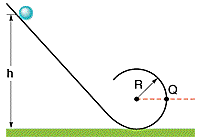# A loop-the-loop track problem

A small solid marble of mass m and radius r will roll without slipping along the loop-the-loop track shown in Fig. 12-34 if it is released from rest somewhere on the straight section of track. For the following answers use m for the mass, r for the radius of the marble, R for the radius of the loop-the-loop and g for the acceleration due to gravity.

Here's the picture:a) a) From what minimum height h above the bottom of the track must the marble be released to ensure that it does not leave the track at the top of the loop? (The radius of the loop-the-loop is R. Assume R>>r.)

Can anyone give me any hints as to how to do this problem, becasue to tell you the truth I don't even know how to start it.

Thanks a lot

## Answers and Replies

Homework Helper
At first I thought this was a "what speed do we have to have in order that centrifugal force will be equal to gravitational force and keep the marble from falling off the track" but you aren't ASKED for speed. You are simply asked for initial height. Since you didn't give any "friction" terms, I'll take it you are ignoring friction and "conservation of energy applies". You simply need to make sure the marble still has SOME speed at the top and that should make the minimum initial height obvious!

(Another hint: If you have a pendulum with no friction and release the bob 1 meter above it's lowest point, how high will it rise on the other side?)

gnome
I don't think so. HallsofIvy's first thought was better.

You aren't asked for speed, but you are asked for the height that will result in the necessary speed.

First, you need an expression for the speed that's needed at the top of the loop such that the force provided by gravity is exactly equal to the centripetal force needed to keep the ball in circular motion (so the ball doesn't fall). For this, use circular motion/Newton's 2nd Law.

THEN, you can use conservation of energy to find the minimum initial height that will achieve the required speed at the top of the loop.

Right, first I needed to find the speed that it has to have at the top of the loop, which was V=Sqr(g(R-r)). Than using conservation of energy I got H to be 2.7 (R-r)...which was the right answer.

Thanks a lot everyone.